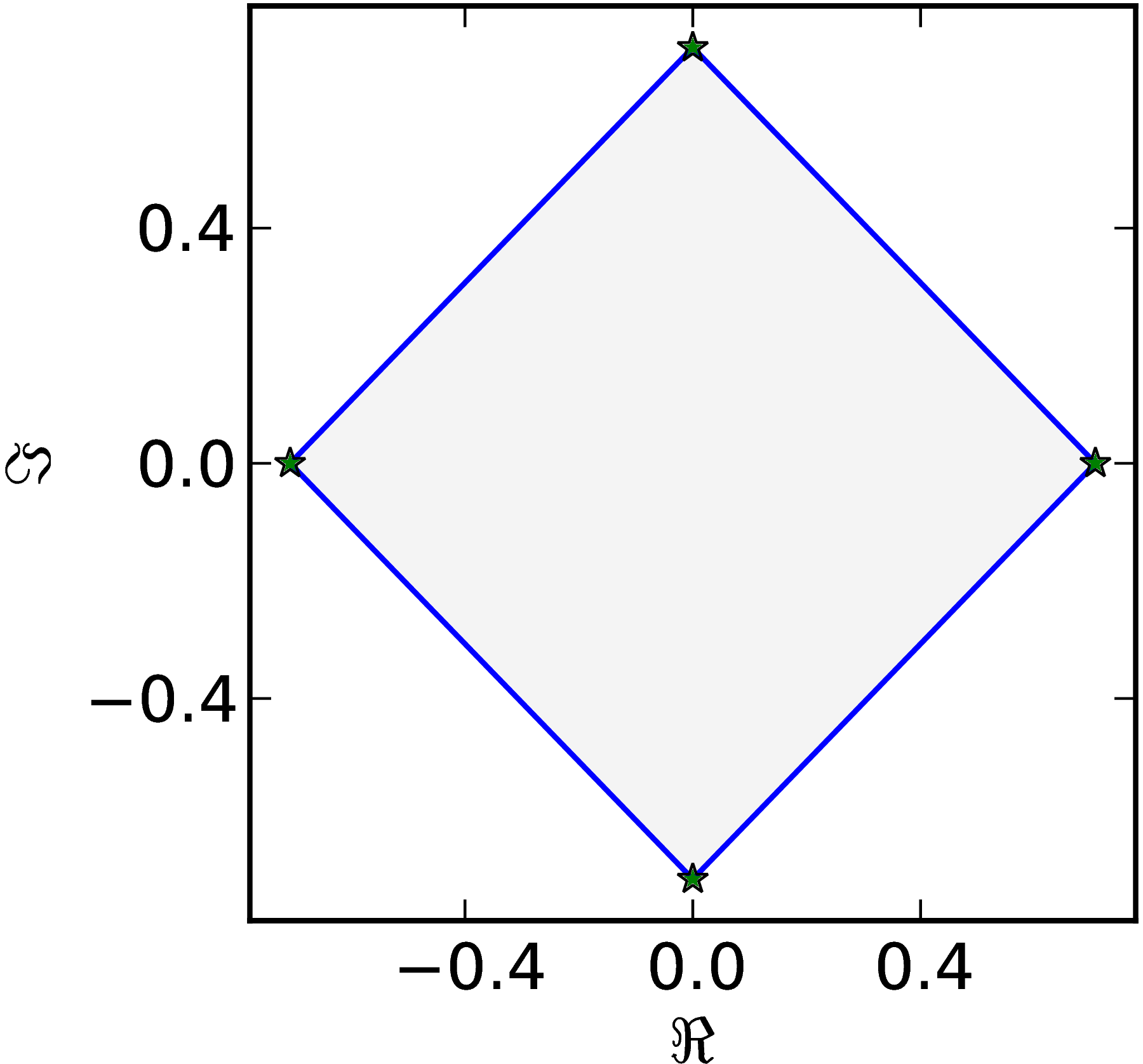### Example 1

A generic matrix $M=\begin{pmatrix} 1 & 1 & 1 & 1\ 0 & \ii & 1 & 1\ 0 & 0 & -1 & 1\ 0 & 0 & 0 & \ii \end{pmatrix}$ has an oval–like numerical range $W (M)$.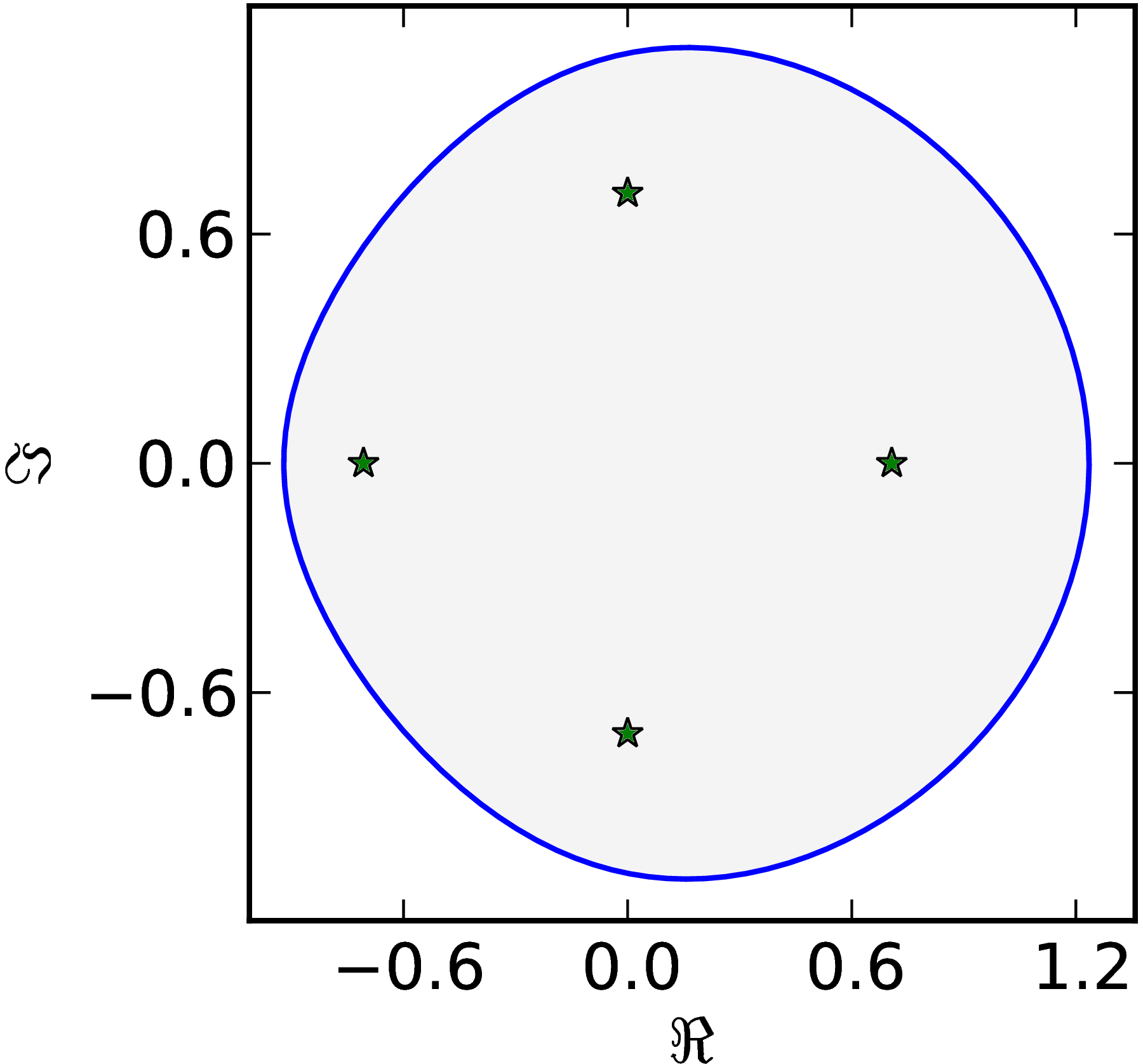### Example 2

The matrix $M=\begin{pmatrix} 1 & 1 & 1 & 1\ 0 & \ii & 1 & 1\ 0 & 0 & -1 & 1\ 0 & 0 & 0 & \ii \end{pmatrix}$ has a numerical range $W (M)$ with one flat part of the boundary $\partial W$.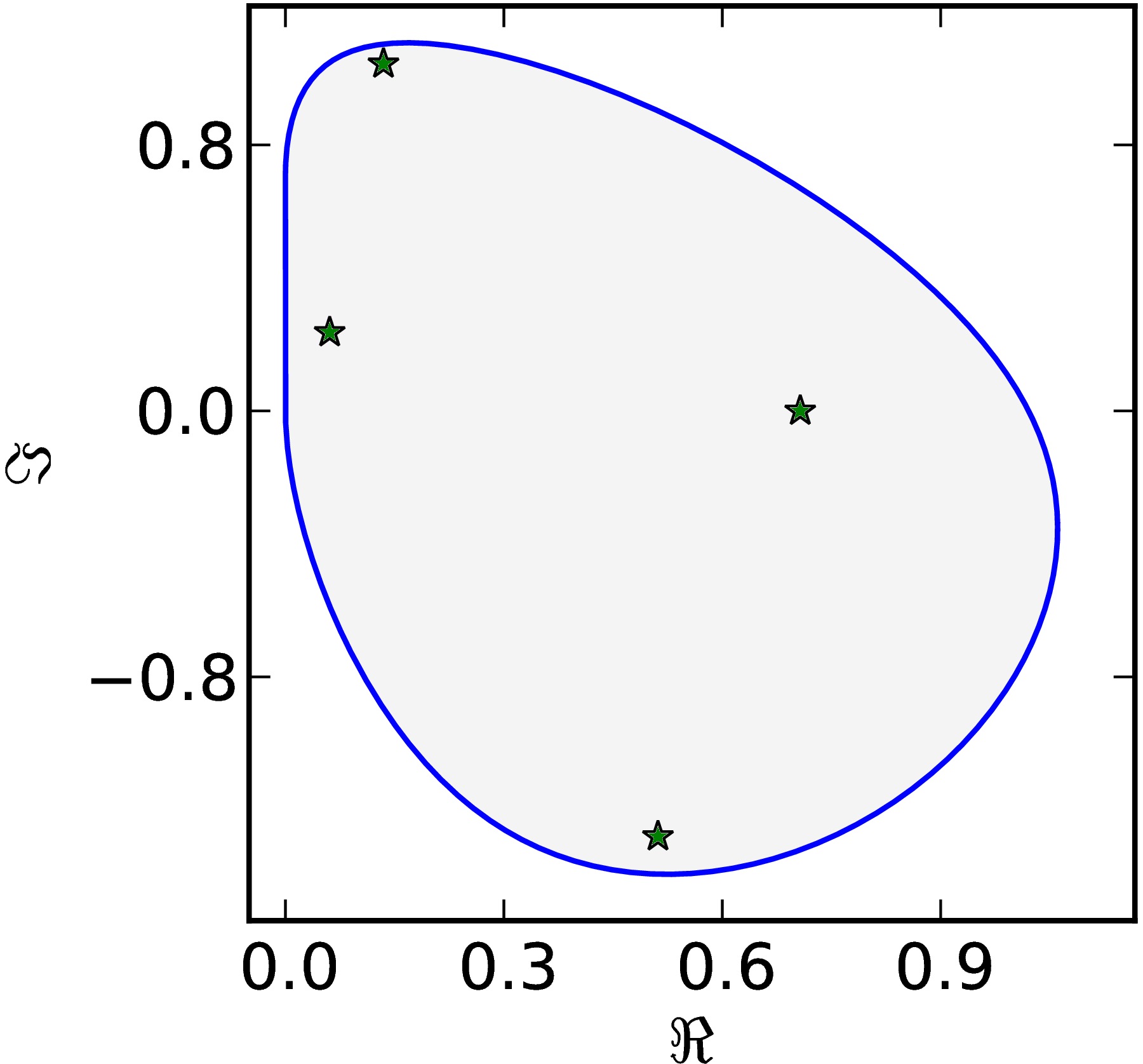### Example 3

The matrix $M=\begin{pmatrix} 1 & 0 & 0 & 1\ 0 & \ii & 0 & 1\ 0 & 0 & -1 & 0\ 0 & 0 & 0 & 1 \end{pmatrix}$ has a numerical range $W (M)$ with two flat parts of of the boundary $\partial W$.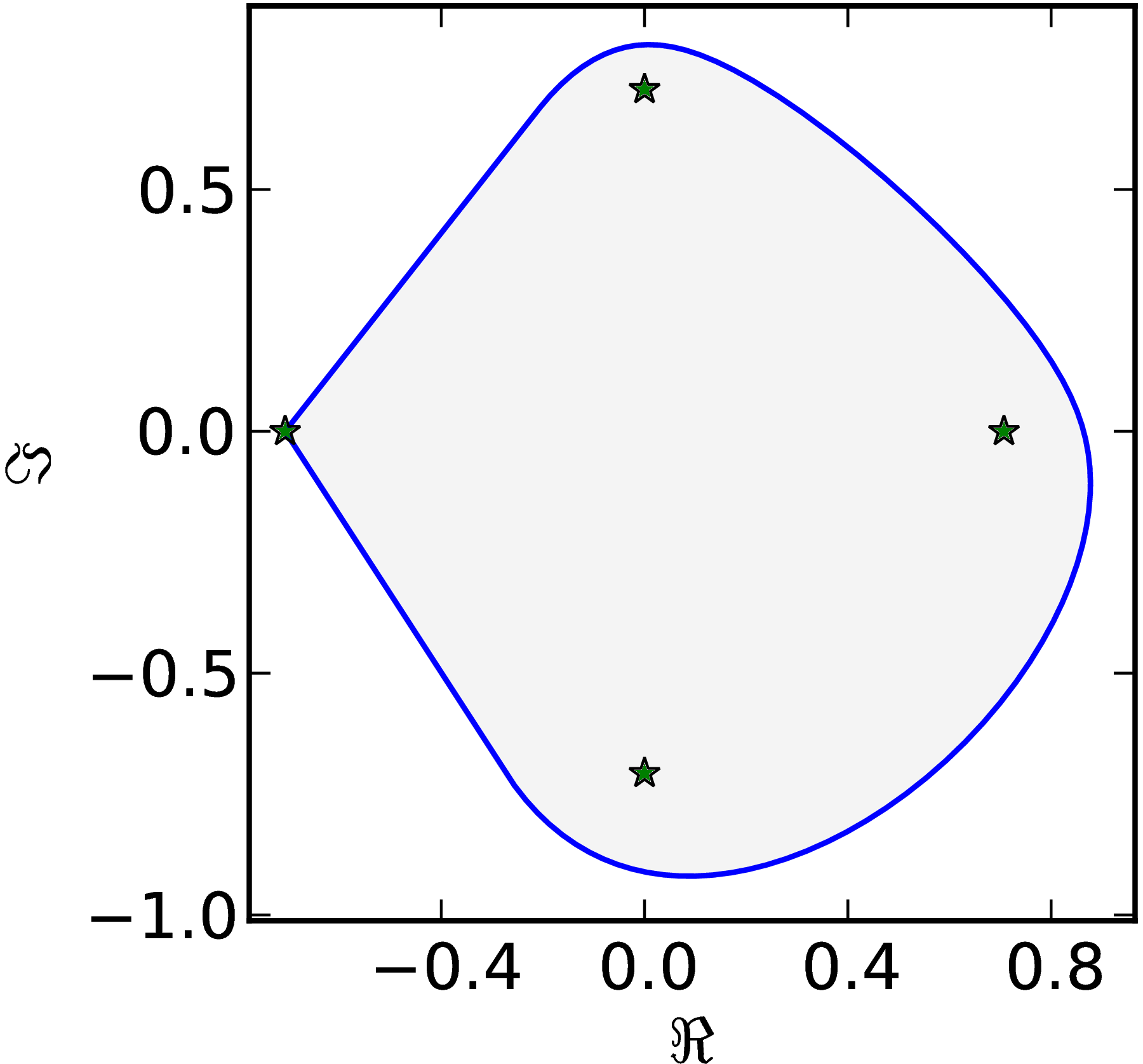### Example 4

The matrix $M=\begin{pmatrix} 1 & 0 & 0 & 1\ 0 & \ii & 1 & 0\ 0 & 0 & -1 & 0\ 0 & 0 & 0 & -\ii \end{pmatrix}$ has a numerical range $W (M)$ with two parallel flat parts of of the boundary $\partial W$.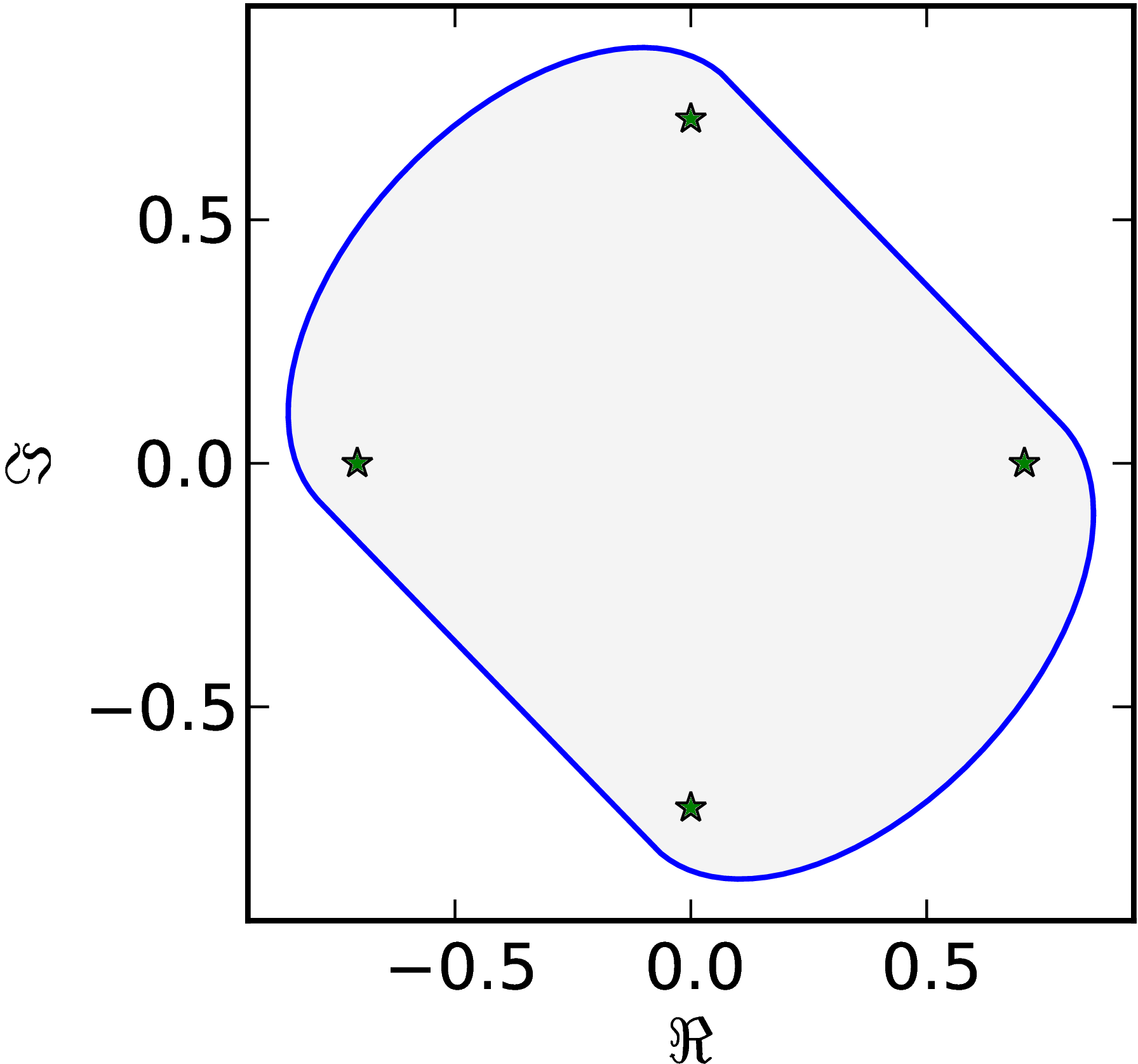### Example 5

The matrix $M=\begin{pmatrix} 1 & 0 & 0 & 1\ 0 & \ii & 0 & 0\ 0 & 0 & -1 & 0\ 0 & 0 & 0 & -\ii \end{pmatrix}$ has a numerical range $W (M)$ with three flat parts of $\partial W$ connected with corners and one oval–like part.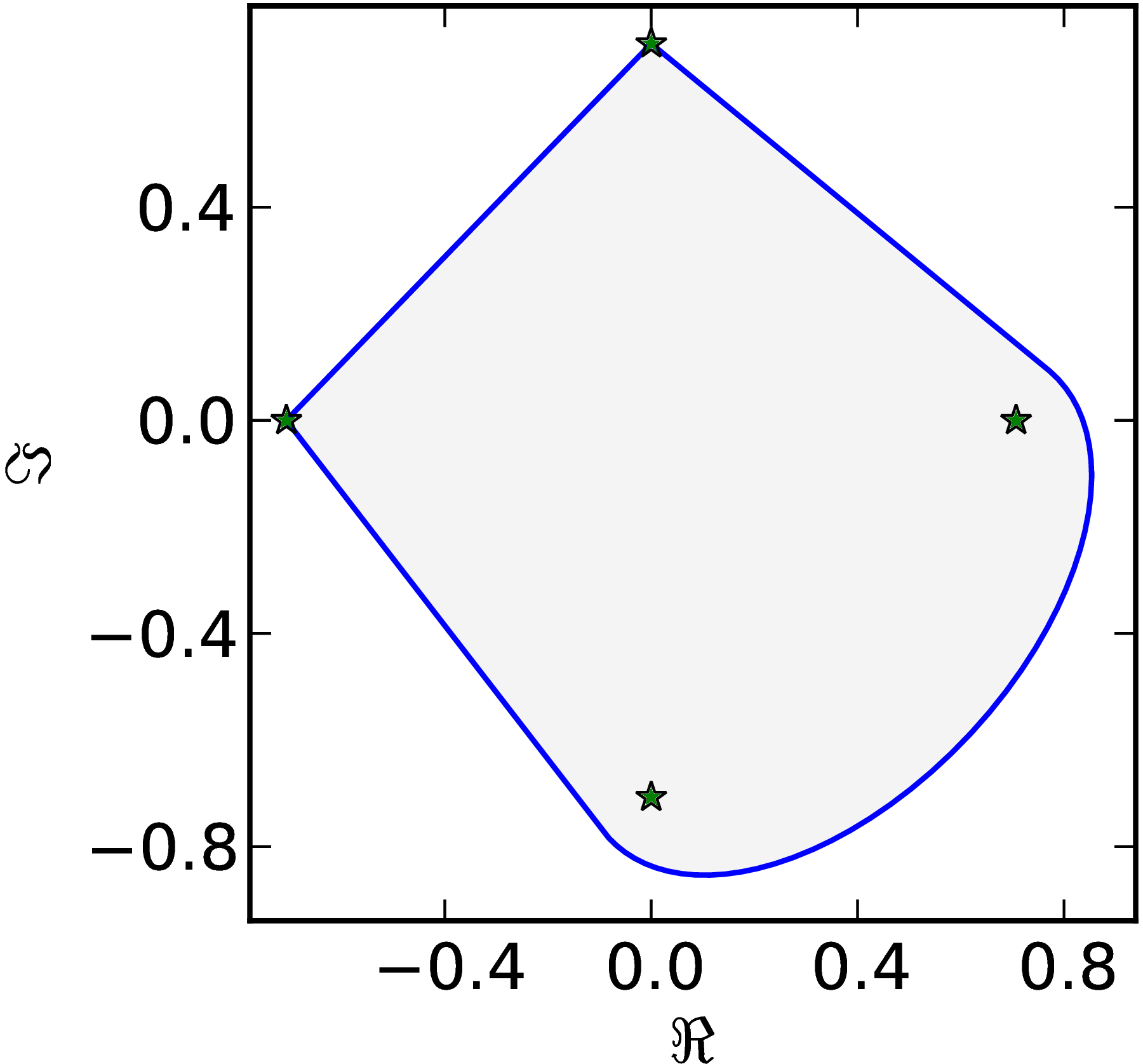### Example 6

The matrix $M=\begin{pmatrix} \ii & 0 & -1 & 0\ 0 & 0 & -1 & 0\ 1 & 1 & 1-\ii & 0\ 0 & 0 & 0 & 1 \end{pmatrix}$ has a numerical range $W (M)$ with three flat parts of $\partial W$ with only one corner and two oval–like parts.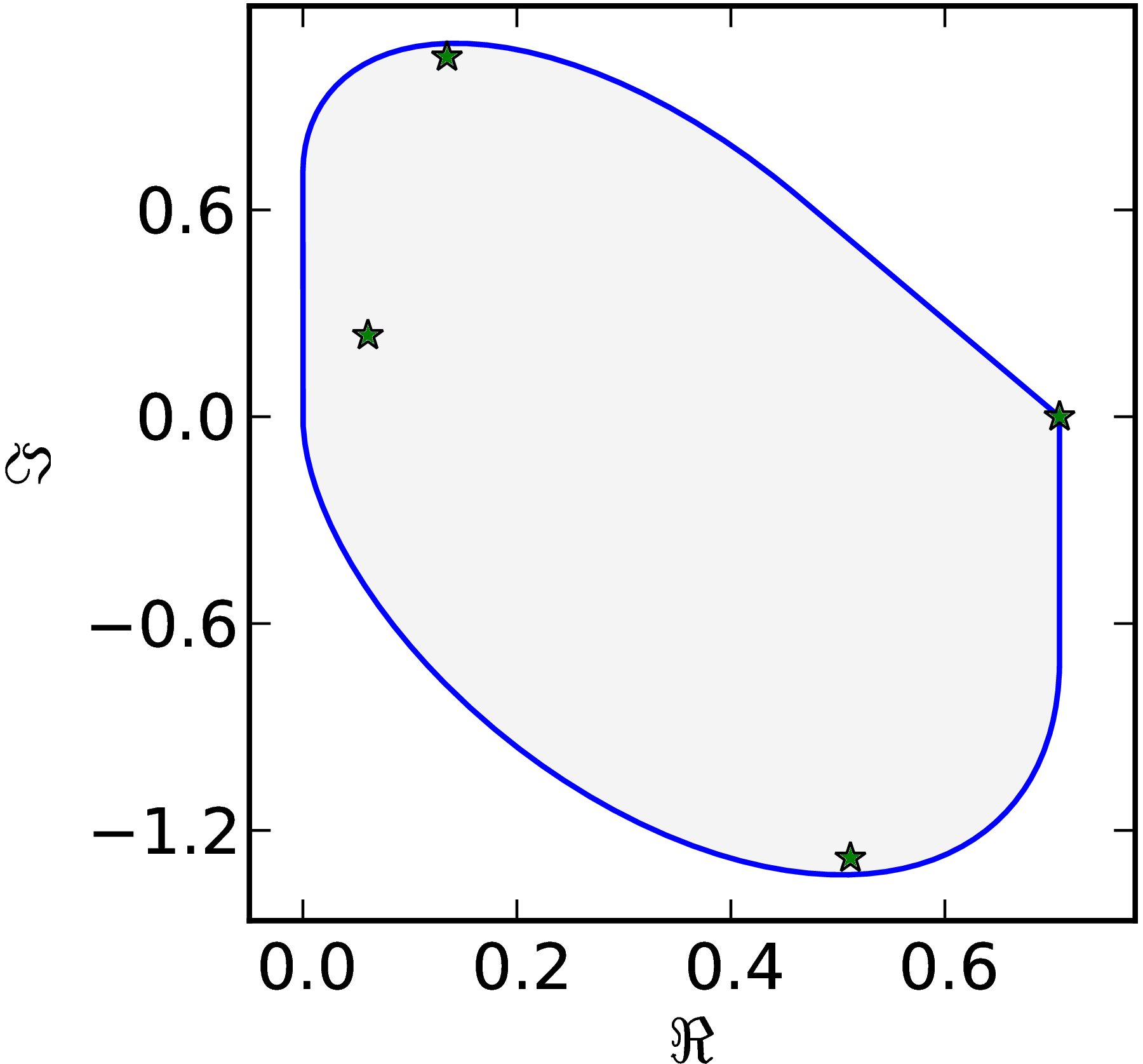### Example 7

The matrix $M=\begin{pmatrix} 1 & 0 & 1 & 0\ 0 & \ii & 0 & 1\ 0 & 0 & -1 & 0\ 0 & 0 & 0 & -\ii \end{pmatrix}$ has a numerical range $W (M)$ with four flat parts of $\partial W$.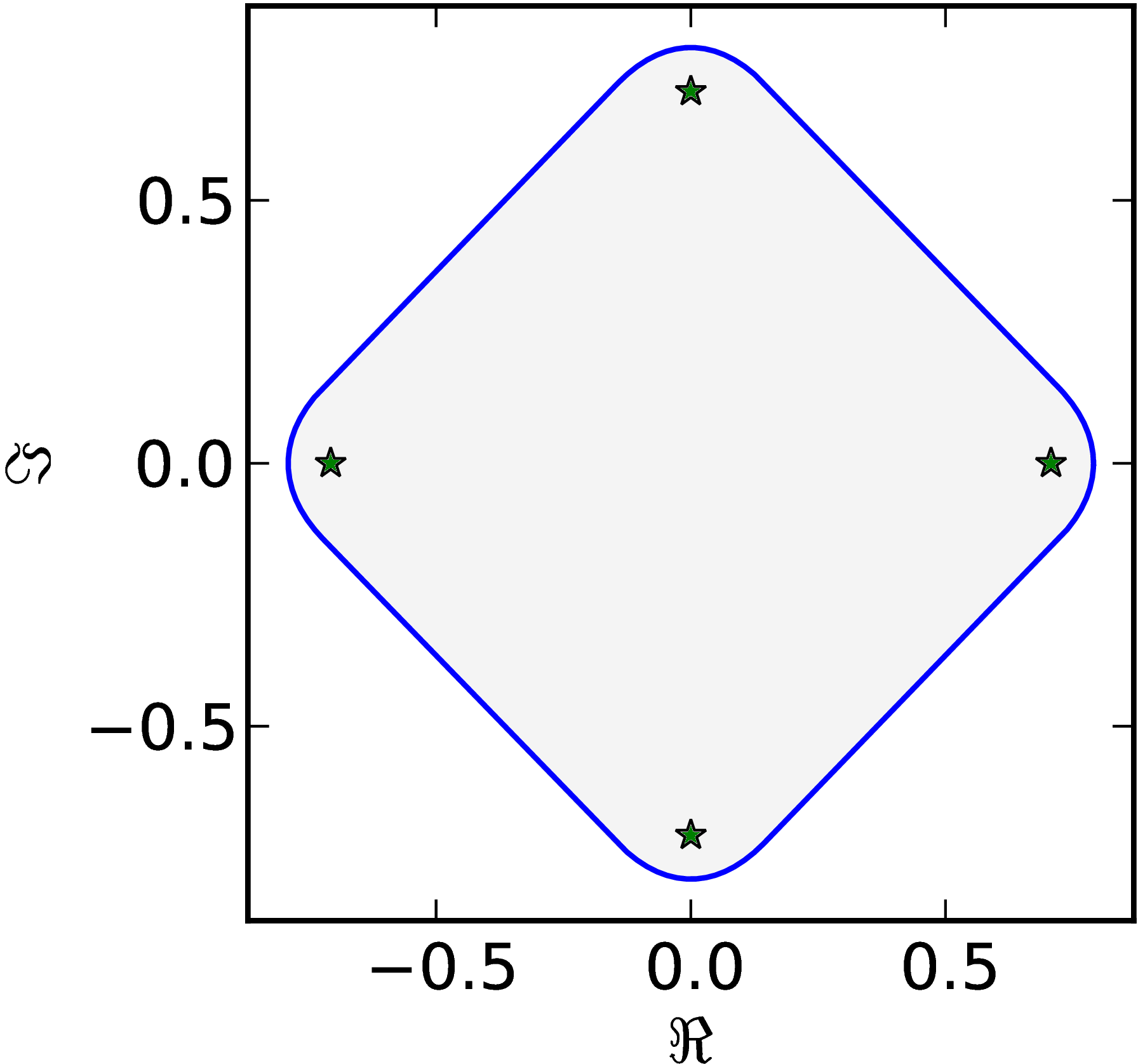### Example 8

The matrix $M=\begin{pmatrix} 1 & 0 & 0 & 0\ 0 & \ii & 0 & 1\ 0 & 0 & -1 & 0\ 0 & 0 & 0 & -\ii \end{pmatrix}$ has a numerical range $W (M)$ pair of flat parts of $\partial W$ connected with a corner connected with two oval–like parts.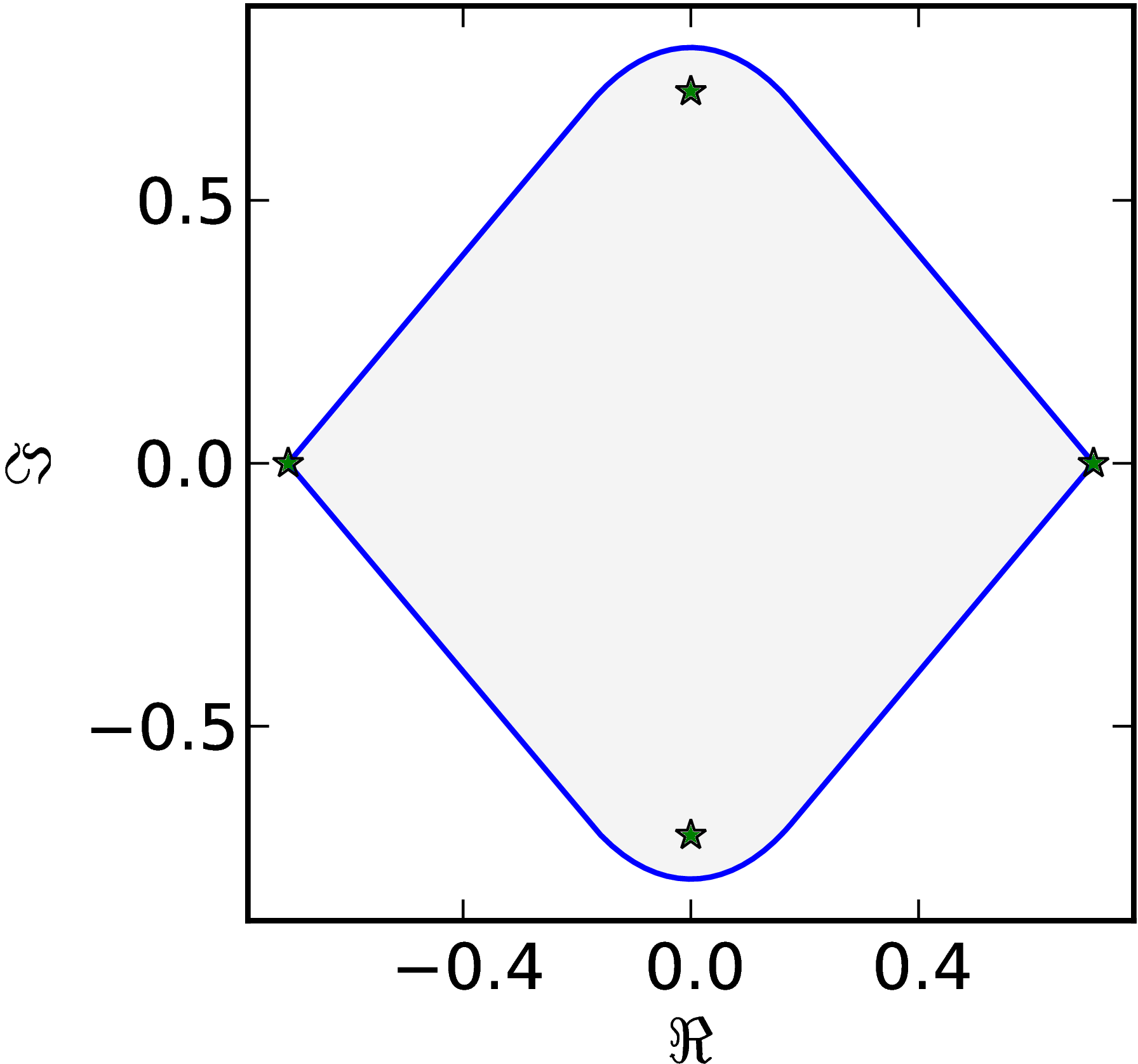### Example 9

The matrix $M=\begin{pmatrix} 1 & 0 & 0 & 0\ 0 & \ii & 0 & 0\ 0 & 0 & -1 & 0\ 0 & 0 & 0 & -\ii \end{pmatrix}$ has a numerical range $W (M)$ equal to the convex hull of eigenvalues.